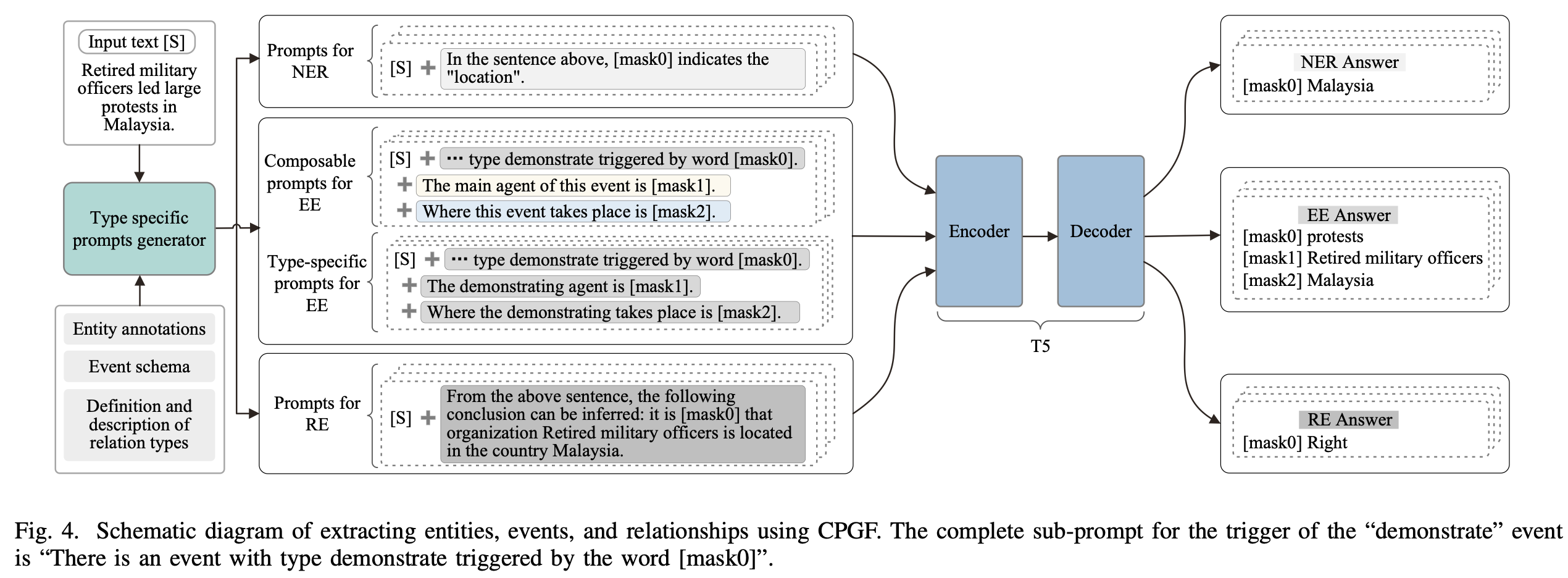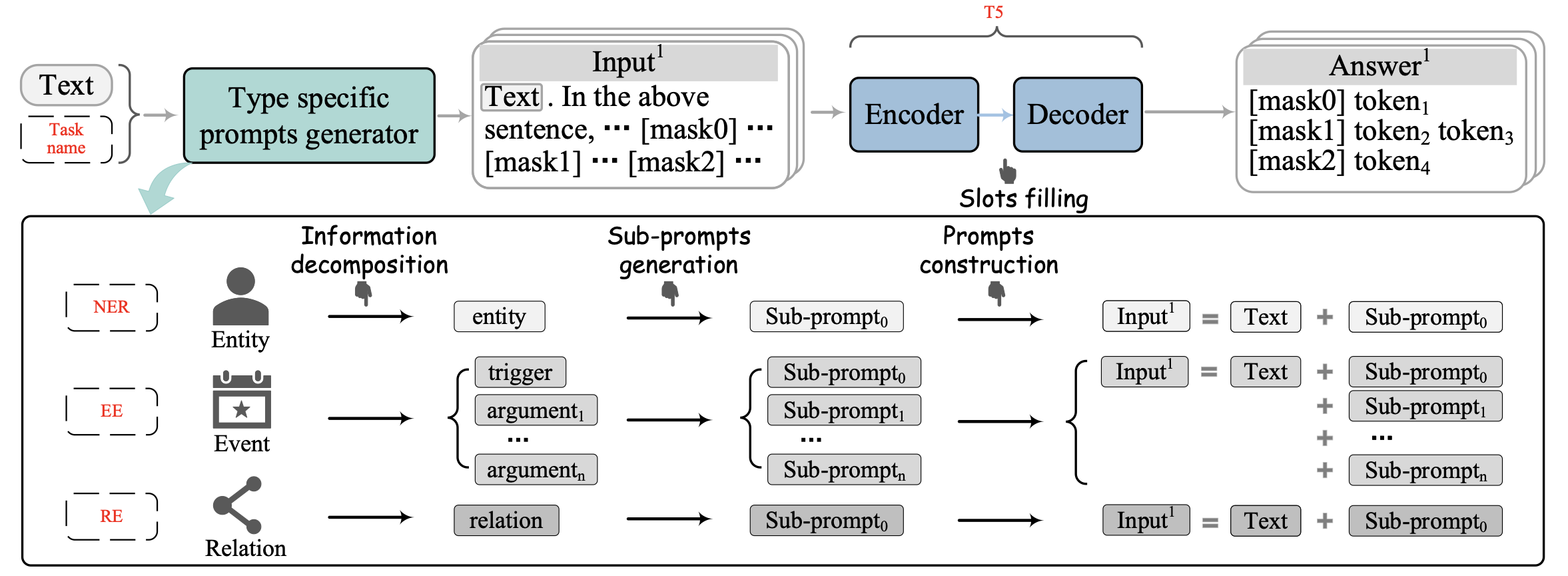## Overview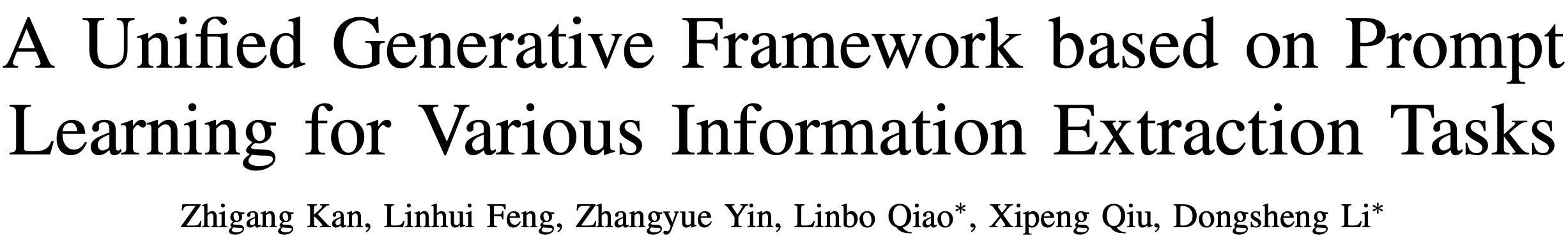## Background

• 采用额外的网络会导致预训练和微调的结构化差异
• 预训练任务和下游任务存在显著差异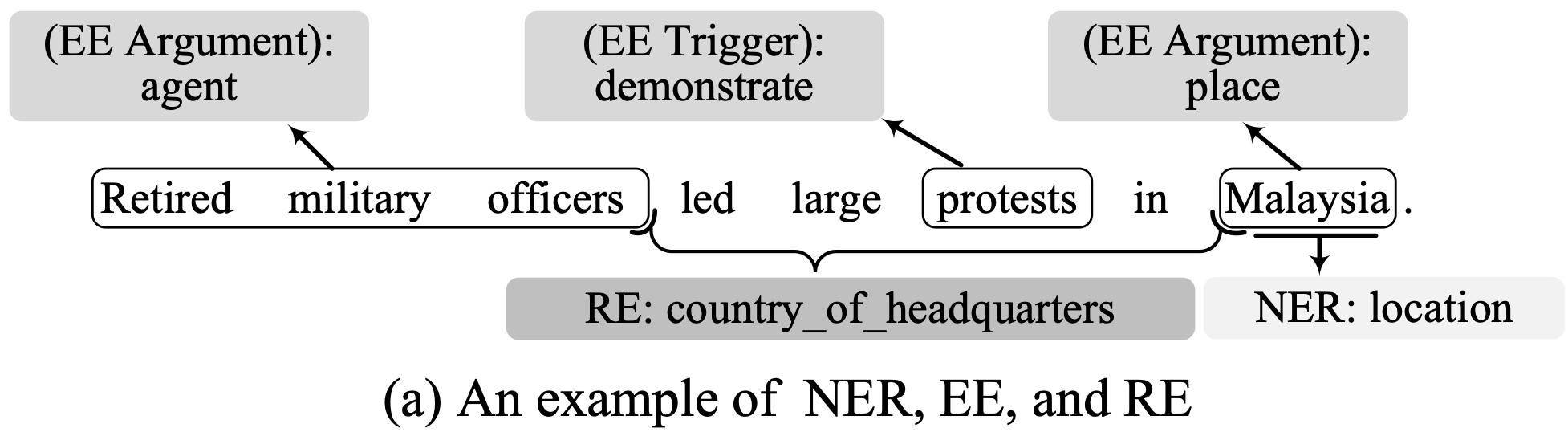prompt范式正是为了修复预训练与微调的不一致，在这种范式下，各种下游任务都转化为类似于预训练的任务。

• LightNER针对NER任务提出了一种生成式框架，其引入了prompt指导的注意力层，在低资源NER任务上取得了很好的成绩，但不能应用到其他IE任务上（例如事件抽取）。

• DEGREE为事件抽取提供了一种模版设计的方法，具体来说，它通过恢复特定类型提示中的受损文本来提取事件。然而，DEGREE通过将所有论据角色的代词集合到一个描述事件发生的短句中，构建了事件类型的特定类型提示。这导致了论据子提示和事件类型之间的密切关联。换句话说，这导致了不同事件类型的论据子提示之间存在相当大的差异。此外，DEGREE也不能应用于其他IE任务（例如关系抽取）。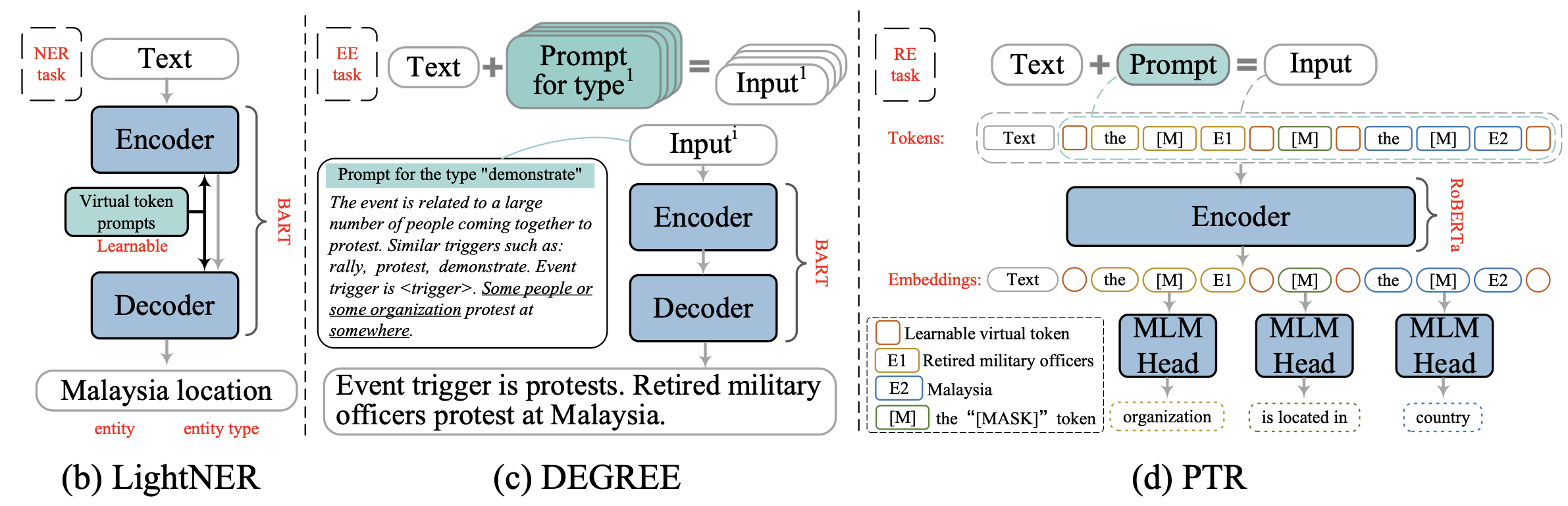• 本文提出了一个基于提示学习范式的新型统一生成框架，用于各种信息抽取任务。
• 本文介绍了一种为复杂任务（如EE）构建独立子提示的方法。此外，为了提高框架在数据稀缺的情况下提取事件的通用能力，为事件提取设计了一种由多个模块化子提示组成的可组合提示。
• 本文提出了一种基于提示的方法，通过判断语义矛盾来实现关系抽取，并为其设计了一个相应的模板。
• 一系列关于EE、NER和RE的实验结果表明，该框架在数据丰富和数据稀缺的情况下都很有效。

## Method

• 信息分解表示将复杂的信息分割成多个片段。
• 子提示生成产生信息片段的子提示。
• 提示构建产生信息类型的特定类型或可组合提示（EE独有）。
• 在填槽阶段，采用T5来预测答案。

### Notation

• 原始文本$$S$$
• 目标信息$$\mathcal{Y}=\{\mathcal{Y}^1,\cdots,\mathcal{Y}^t\}$$
• $$\mathcal{Y}^j$$表示类型$$j$$的待提取信息，$$t$$为类型总数
• 对于EE，$$\mathcal{Y}^j$$可能包含多个元素，如事件类型和论元角色
• 提示$$Pr=\{Pr^1,\cdots,Pr^t\}$$

### Sub-Prompts Generation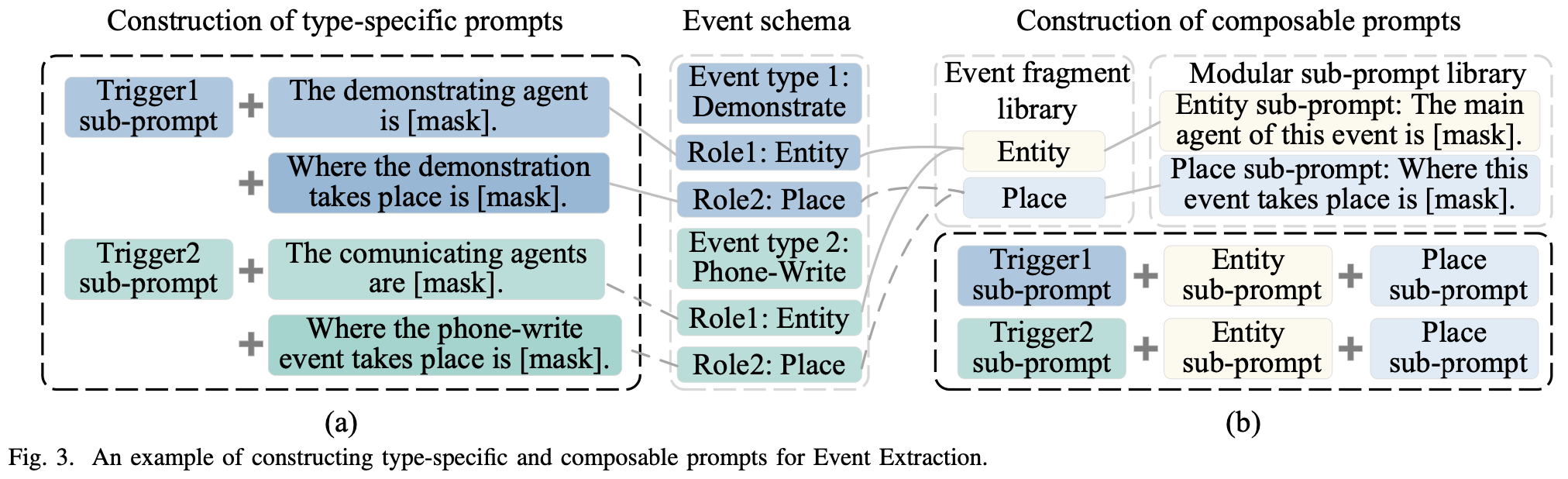### Prompts Construction

$$j$$种信息类型的提示是通过连接原始文本和子提示来构建的： \begin{align} \mathcal{S}_{\text{prompt}}^j &= f_{\text{prompt}}(S) \\ &= S \mathop{\Vert} Pr^j \end{align} 其中$$\mathop\Vert$$表示拼接两段文本，$$Pr^j$$表示组合目标信息中所有相关元素的子提示： $Pr^j = \mathop{\Vert}_{i=1}^n p_i^j$ 对于NER和RE，$$n$$始终为1。对于EE，$$S^j_{\text{promt}}$$被称为特定类型的prompt，如果用来组成的提示语是依赖于类型的，因为这个提示语的每个部分都与信息类型有关。由模块化子提示组成的提示被命名为 "可组合提示"。